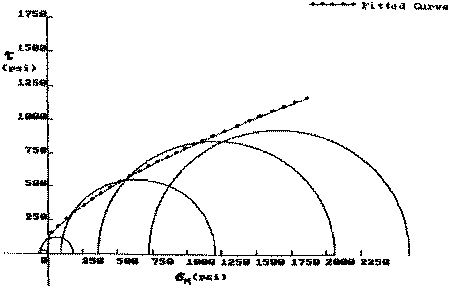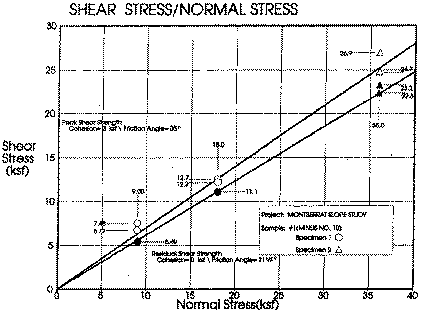### Strength of wall rock of English's Crater,Soufriere Hills Volcano, Montserrat

B. Voight

Department of Geosciences, Penn State University

Results are summarized here for the rock test program, on samples collected by BV in Spring 1996. Results include bulk specific gravity (SG), tensile strength (T), and the Coulomb parameters cohesion (C) and internal friction angle (PHI). Strength values are in pounds force per square inch (psi), angles are degrees. Conversions for units are as follows: 1 psi = 0.0703 kg f/sq cm = 6.895 Pa.

For Gages Wall altered dacite, SG = 1.84, T = 60; nominally, C = 250, PHI = 32. The latter Coulomb values are approximations valid for a portion of the normal stress range. The actual experimental data from triaxial compression tests indicate a curved strength distribution (Figure 1). These data may be fitted by hand using a French curve, or by a computer algorithm for a non-linear envelope. A piece-wise linear fit to the data by hand can provide reasonable Coulomb parameter approximations for different ranges of normal stress.Figure 1: Gages Wall altered dacite. Shear stress (t) v.s. normal stress (sigma) diagram, with individual triaxial shear test data plotted as Mohr circles that indicate maximum and minimum stresses at failure. Arbitrary curved strength envelope fitted to test data. Linear (coulomb) approximations are discussed in the text.

For Galways Wall altered dacite, sample 1, SG = 1.98, T = 175; for normal stresses above 300 psi, C = 530, PHI = 31. As described above, the test data indicate a curved envelope, so that the linear approximations of C,T above overestimate the strength at low confining pressures. Considering all the data yields the linear fit C=317, PHI =33 ; this set of parameters underestimates strength at intermediate confining pressures. As above, a piece-wise linear fit can provide reasonable Coulomb parameter approximations for different ranges of normal stress.

Strengths reported here are upper bounds for material represented by the samples; values could be reduced to account for sample diameter (1 inch) and rock mass jointing. This is a matter of geotechnical judgment, but lower bound strength values are provided by direct shear strength tests on disaggregated crater wall material. The strengths are given by Coulomb parameters C = 0, and PHI. In this case the test data are accurately portrayed by linear envelopes.

For Gages Wall disaggregated materials, texturally a silty sand with gravel, peak strength with moderate compaction is PHI = 35 (Figure 2) and the residual displacement strength is PHI = 31.5 (degrees). For Galways Wall disaggregated material, also silty sand with gravel, peak strength PHI is 35.5, and residual strength = 30.5. Thus for both sites a single nominal set of parameters seems appropriate, viz. C = 0 , PHI = 35 (peak) and C = 0, PHI = 31 (residual). These parameters are appropriate for disaggregated material or uncemented joints within the tested range of normal stresses to 36,000 pounds per square foot.Figure 2: Gages Wall disaggregated material. Shear stress vs normal stress diagram, for direct shear tests. Linear strength envelopes plotted for peak and residual values. Note stresses are in kfs units, kips per square foot; 1 kip = 1000 pounds of force.